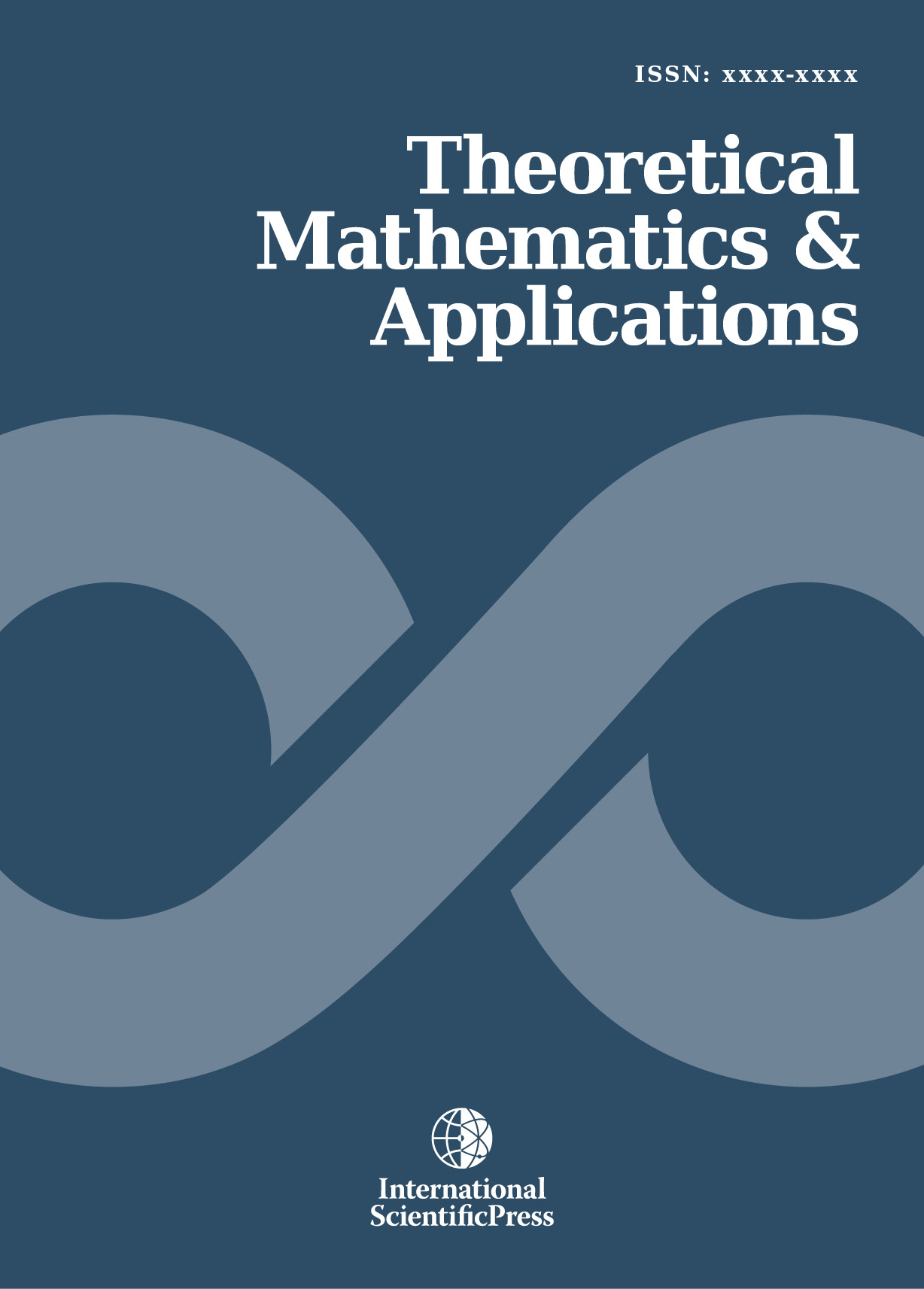# Theoretical Mathematics & Applications

#### On harmonic analysis of spherical convolutions on semisimple Lie groups

•[ Download ]
• Times downloaded: 911
•                                                    Abstract

This paper contains a non-trivial generalization of the Harish-Chandra transforms on a connected semisimple Lie group G, with finite center, into what we term spherical convolutions. Among other results we show that its integral over the collection of bounded spherical functions at the identity element e ε G is a weighted Fourier transforms of the Abel transform at 0. Being a function on G, the restriction of this integral of its spherical Fourier transforms to the positive-definite spherical functions is then shown to be (the non-zero constant multiple of) a positive-definite distribution on G, which is tempered and invariant on G = SL(2,R). These results suggest the consideration of a calculus on the Schwartz algebras of spherical functions. The Plancherel measure of the spherical convolutions is also explicitly computed.ISSN: 1792-9687 (Print)
1792-9709 (Online)